# AP Physics 2 Practice Test 1

### Test Information10 questions18 minutes

1. What happens to the image formed by a concave mirror as the object is moved from far away to near the focal point?

2.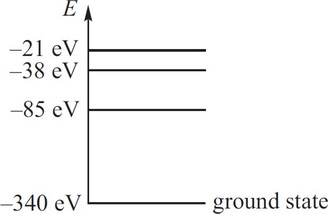The diagram above (not drawn to scale) gives the first few electron energy levels within a single-electron atom. Which of the following gives the energy of a photon that could NOT be emitted by this atom during an electron transition?

3. During an isothermal expansion, a confined ideal gas does 150 J of work against its surroundings. Which of the following describes the heat transfer during this process?

4.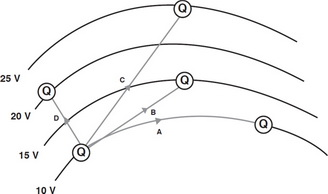The figure above shows the equipotential lines a positive charge Q moves through. Along which path is the most work done on the charge?

5. An object of volume 2 × 10-3 m3 and weight 6 N is placed into a tank of water, where it floats. What percentage of the object's volume is above the surface of the water?

6.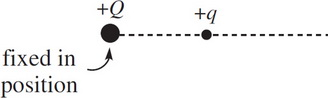The figure above shows two positively charged particles. The +Q charge is fixed in position, and the +Q charge is brought close to +Q and released from rest. Which of the following graphs best depicts the acceleration of the +Q charge as a function of its distance Q from +Q ?

7. Each of the following particles is projected with the same speed into a uniform magnetic field B such that the particle's initial velocity is perpendicular to B. Which one would move in a circular path with the largest radius?

8.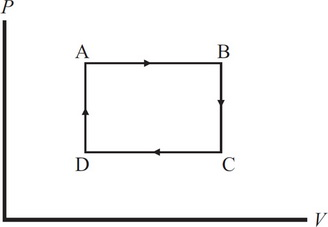Which statement correctly characterizes the work done on the gas during the ABCA cycle shown in the above P-V diagram?

9. How much current does a 60 W light bulb draw if it operates at a voltage of 120 V ?

10. Question below refers to the following plane diagram. The figure shows four point charges arranged at the corners of a square with point B as its center.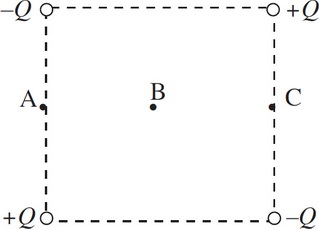At which of the three points shown in the figure would a positive charge experience no net force?